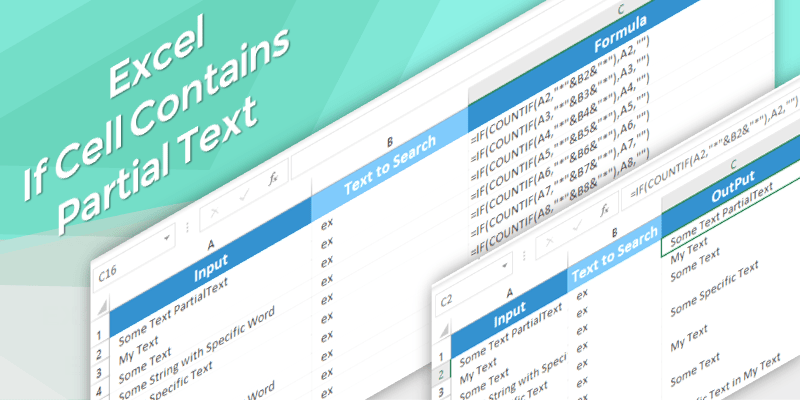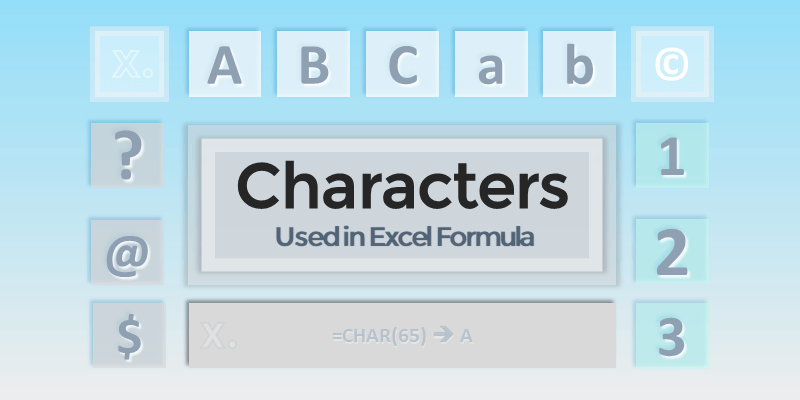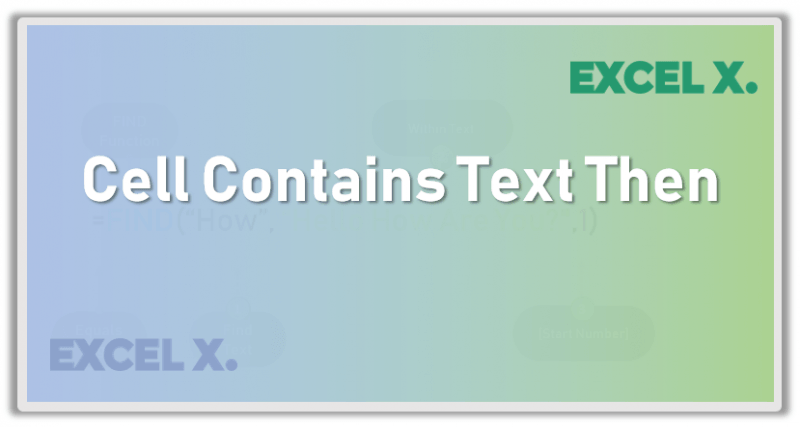•Excel if cell contains partial text
Gallery

## Excel if cell contains partial text

Here is the Excel formula to Check If Cell Contains Partial Text. You can se this formula to determine the cell contains partial text and

## Check If Range of Cells Contains Specific Text

Below is the Excel formula to Check If Range of Cells Contains Specific Text in Excel. You can check one or range of cells for

## If Cell Contains Specific Text Then Return Value

Here is the the Excel formula to check if cell contains specific text then return value. Often, we need to search for a text in

•Operators in Excel
Gallery

## Operators in Excel

Operators in Excel helps to perform verity of operations in Excel Calculations. Excel Operators are very useful in creating Formulas and Expressions. We can divide

•Characters in Excel
Gallery

## Characters in Excel

What are Characters in Excel? Characters in Excel are the letters, numbers and  special characters. Each character represented with a code and saved with a

## Symbols Used in Excel Formula

Here are the important symbols used in Excel Formulas. Each of these special characters have used for different purpose in Excel. Let us see complete

## Display Cell Contents in Another Cell in Excel

Very often we reference the data of a Cell in another Cell in Excel. We can use equals to sign (=) then enter the

•Excel If Cell Contains Text
Gallery

## Excel If Cell Contains Text

Excel If Cell Contains Text Then Formula helps you to return the output when a cell have any text or a specific text. You can

## Excel Formula If Cell Contains Text Then Return Value in Another Cell

Here is the Excel Formula If Cell Contains Text Then to Return Value in Another Cell. This is the very useful formula when we deal